# Wavelet-based Statistical and Mathematical Analysis of Spread of COVID-19

Short Communication

# Wavelet-based Statistical and Mathematical Analysis of Spread of COVID-19

### Samreen Fatima*, Mehwish Shafi Khan, Yumna Sajid

Adv. life sci., vol. 8, no. 3, pp. 333-338, December 2021
*Corresponding Author: Samreen Fatima (Email: sf_qudduss@hotmail.com)
Authors' Affiliations

Department of Statistics, University of Karachi – Pakistan
[Date Received: 07/05/2020; Date Revised: 26/09/2021; Date Published: 31/12/2021]

Abstract

The outbreak of coronavirus-19 (NCoV-19) has developed a universal crisis due to high rate of infection and mortality. Therefore, the researchers are using various available methods to study the pattern of spread of COVID-19 which will help in planning to control the disease and to manage the health care resources. This study compares Autoregressive Integrated Moving Average (ARIMA) (statistical), Logistic, Gompertz (mathematical) and their hybrid using Wavelet-based Forecast (WBF) models to model and predict the number of confirmed cases of COVID-19. The study area includes the countries: Iran, Italy, Pakistan, Saudi Arabia, USA, UK and Canada. Moreover, root mean squares error (RMSE) is used to compare the performance of studied models. Empirical analysis shows that confirmed cases could be adequately modelled using ARIMA and ARIMA-WBF for all the countries under consideration. However, for future prediction significance of the models varies region to region.

Keywords: COVID-19; ARIMA; Logistic; Gompertz; Wavelet-based-forecast

Rresearchers from all over the world from various fields are engaged in studying the pattern of the COVID-19 outbreak. Concerning the modeling and forecasting of COVID-19 cases, a large number of studies have been carried out employing the existing mathematical or statistical models during the last few months including other epidemic variables which may cause rapid increase in pandemic [1-6].

Moreover, statistical models including short-term/long-term, box Jenkins Autoregressive Integrated Moving Average (ARIMA) model, time series generalized linear mixed models (GLMM), Generalized logistic model have been applied to study the pattern and growth of COVID-19 [3,7-12]. Classical mathematical models: Susceptible Infectious and Recovered (SIR) and Susceptible, Exposed, Infectious and Recovered (SEIR) models [12-14] along with its modifications eSIR have been used to model the current outbreak of COVID-19 . In addition to these, transmission risk and prediction of the peak time of COVID-19 has also been done via mathematical model based on the clinical evolution .

As the COVID-19 data was available in limited quantity due to its early stage therefore, advanced deep learning methods might over fit the training set . However, these models have different approach subject to geographical location, dimension of variables and time period. Therefore, it is important to develop country specific model which can model COVID-19 outbreaks precisely.

This study utilizes mathematical and statistical techniques for confirmed COVID-19 cases in two parts.  In the first part, statistical model ARIMA and mathematical models: Logistic and Gompertz are employed. Whereas their wavelet-based hybrid models are developed in the second part. The daily confirmed cases data are selected for the countries: Pakistan, Iran, Italy, Saudi Arabia, UK, USA and Canada, which belong to different geographical location and different economic background. Among all considered countries, Canada, Italy, UK and USA are highly developed regions containing good health facilities and belong to highest income group of countries. However, Pakistan and Iran falling in the category of developing countries holding poor health facilities. Furthermore, to assess the performance of the studied models RMSE (root mean squares error) criterion is used.

Autoregressive Integrated Moving Average

ARIMA (p, d, q) model is the linear combination of Autoregressive (AR(p)) and Moving Average (MA(q)) process. Where, p and q represent the order of AR and MA processes and d is the number of times that the integrated process must be differenced to make a time-series stationary. ARIMA (p, d, q) model is described as follows: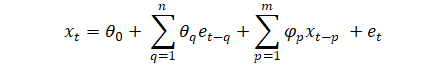Where, ‘xt’ is time series, xt-p’s are their lag values and et is error having mean zero and constant variance satisfy the condition of i.i.d.  Moreover, ji and qj are the parameters of the model which are determined either by the least square method or method of maximum likelihood.

Logistic Growth model

Verhulst, a mathematician improved the Malthusian population growth model proposed in 1977 and named it as Logistic function . It is widely used in various fields from biology to computer science. In this study three parameters based logistic model is used to fit the COVID-19 data of the total confirmed cases described as follows: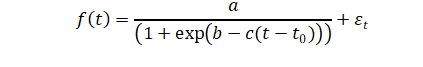…… (1)

Where, f(t) is the number of confirmed cases at time ‘t’, constant ‘c’ is the continuous growth rate determining the rate of infection spread, ‘a’ is the maximum number of confirmed cases b is the fitting coefficients and to is the time when the first case occurred.

Gompertz model

The Gompertz function or Gompertz model was originally proposed by Gompertz for modeling of an infection spread . It belongs to the family of sigmoid function which is categorized by a slow growth rate curve at the beginning and at the end of a given time period whereas the function grows sharply in the middle of the period. Mathematically, Gompertz function can be expressed as: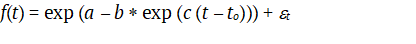… … (2)

Where, ‘t’ is time and to is the time when the first case occurred, ‘a’ is the predicted maximum of confirmed cases and ‘b’ and ‘c’ are slopes.

The parameters of the Logistic and Gompertz models are determined by nonlinear least square method (NLSM) which minimizes the sum of squares of error.

Wavelet Based Forecast

WBF is used to extract information via signal decomposition in the domain of time and frequency . Discrete Wavelet Transform (DWT) , with initial resolution of one signal as K can be defined as , where, W(t) is scaling function and w(t) is the family of wavelets (see  for more detail). In this study, maximal overlap discrete wavelet transform (MODWT) is applied instead of DWT due to its limitations: requirement of sample size of multiple of 2 and output of DWT is highly dependent of origin of the signal that would be affected upon a slight shift in origin [23,24]. The MODWT pyramid algorithm  generates wavelet coefficients (r j,n) and the scaling coefficients (s j,n) from (s j-1,n) defined as: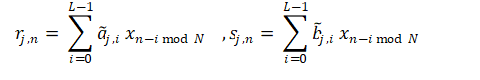Above expression is for periodic filter operations of the actual series (xn) with filters:  and . Where, N is length of time series and n varies from 0 to N-1, aj and bj are coefficients of scaling and wavelet filters, respectively. Moreover, the original signals can be recovered by applying inverse pyramid algorithm :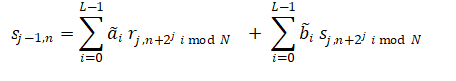Wavelet Based Hybrid Models

Here ARIMA-WBF is explained, and a similar approach is applied on all hybrid models (Logistic-WBF and Gompertz-WBF) as discussed here for ARIMA-WBF. A hybridization of ARIMA and WBF model has been opted to minimize the biases of the individual models . In contrast to ARIMA, WBF are more likely to deal with non-stationary time series data. Furthermore, non-stationarity of pandemic data motivates the selection of WBF for modelling the data [26,27]. In this study, ARIMA-WBF has been applied steps-wise as explained by Gosh . Firstly, ARIMA model is applied to model the time series of length  and  numbers of forecast are done on the basis of model obtained. Secondly, the non-stationary residual series of length  generated during previous step is further passed to WBF model. Moreover, WBF acquire in-sample residual predictions and provide out-sample forecast as well using decomposition levels as WL = log (n) and boundary as ‘periodic’. Finally, forecasts obtained from ARIMA and WBF are combined for both in-sample and out-sample data to achieve hybridized prediction.

Data Analysis

Univariate daily time-series data of COVID-19 for the time period 1st January 2020–4th May 2021 are collected from https://ourworldindata.org/coronavirus-source-data. The total number of confirmed COVID-19 cases per million and total number of deaths per million populations (now onwards referred as confirmed cases and death cases respectively) of seven countries from different geographical locations including: Canada, Iran, Italy, Pakistan, Saudi Arabia, USA and UK are considered in this study.

From basic statistics (graph\standard deviation), it is noted that increase in number of confirmed cases in a particular month leads to increase in mortality rate in the following month. Therefore, to study the relationship between the numbers of confirmed cases and death cases correlation and descriptive statistics are calculated for the countries under consideration (Table1). However, only confirmed cases are analyzed and modeled for future prediction via statistical and mathematical models. Moreover, the data is divided into model building from 1st January to 24th February, 2021 whereas validation period consists of 25th February, 2021 to 4th May 2021.

Among all the countries under analysis, average number of confirmed cases are high in US followed by UK, Italy, Canada, Iran, Saudi Arabia and Pakistan over the considered time period. Moreover, the average number of death cases are high in UK followed by Italy, US, Iran, Canada, Saudi Arabia and Pakistan. The Standard Deviation (Std. Dev) of confirmed cases is high in US followed by UK, Italy, Canada, Iran, Saudi Arabia and Pakistan. The Std. Dev of death cases is high in UK followed by Italy, US, Iran, Canada, Saudi Arabia and Pakistan. Overall, Pakistan and Saudi Arabia are less affected by COVID-19 among all considered countries on the basis of confirmed and death cases. However, the correlations between the confirmed cases and death cases are high in all the countries, (Table 2). To fit the logistic and Gompertz functions on confirmed cases, Mathematica routine using the minimum least squares method is compiled.

For the statistical model ARIMA, ADF test is used to check the stationarity of the data. Moreover, auto.arima() is applied for acquiring suitable ARIMA model based on minimum AIC and BIC criterion including the condition of significance of parameter at 5% level under R environment. In the next step hybrid models: ARIMA-WBF, Logistic-WBF(GL-WBF) and Gompertz-WBF(GUP-WBF) are applied on the confirmed cases to enhance the prediction performance. Based on RMSE, ARIMA is found at the top whereas ARIMA-WBF is on the second number for in sample forecast for all the countries. However, in case of out sample both ARIMA and ARIMA-WBF found suitable for Pakistan, Saudi-Arabia, Italy and US.  Whereas for the Iran and Canada GL-WBF and for US has logistic curve give minimum out sample forecast.

Moreover, Fig. (1 to 7) show the plots of actual and fitted data along with the forecasted one. Furthermore, during model building period it is observed that actual values are overlapped by most of the modelled fitted values except for ARIMA for Saudi Arabia and Logistic and Gompertz-WBF in case of Iran, Italy, UK and USA, respectively. However, during out-sample forecasting each model either underestimate or overestimate the prediction which has already been discussed via RMSE.

In the above fig 1, it is observed that Gompertz and Logistic models poorly estimate the in-sample and out-sample forecast. Fig 2 shows that in-sample and out-sample forecast is fairly mapped by Gompertz-WBF, Logistic and Logistic-WBF. Whereas ARIMA and Gompertz poorly captured the in-sample as well as out-sample forecast. Fig 3 shows that the in-sample forecast fairly captured by almost all models except Gompertz -WBF. Whereas all the models over-predict in out-sample forecast. Fig 4 shows that the in-sample forecast fairly captured by almost all models except Gompertz. Whereas out-sample forecast over-predict by Gompertz-WBF, Logistic and Gompertz.

Figure 5 below shows that in-sample forecast poorly captured by Logistic and Gompertz. Whereas out-sample forecast is poorly captured by all models except Logistic-WBF. Fig 6 shows that the in-sample forecast captured by all models are good fitted and out-sample forecast are over predicted by Gompertz-WBF and Logistic model. Graph of Canada shows that the in-sample forecast decently captured by ARIMA, Arima-WBF, Logistic-WBF and Gompertz-WBF. Whereas out-sample forecast captured by Arima-WBF, Logistic and Gompertz are over predicted.

Figures & Tables

In this paper, statistical and mathematical models are applied with their hybrid using WBF including: ARIMA, Logistic, Gompertz, ARIMA-WBF, Logistic-WBF and Gompertz-WBF on COVID 19 confirmed cases. COVID-19 data is considered from 31st December, 2019 to 4th May, 2021 for Pakistan, Saudi Arabia, Iran, Italy, United Kingdom, Canada and United States of America. Moreover, above models being data driven behave in different manner in different regions. Therefore, no specific model could be ranked as best or worst however for a particular region and period the model could be chosen smartly on the basis of statistical and graphical analysis. From both visual and statistical analysis, it is found that ARIMA was able to capture in-sample forecast adequately in all countries. In contrast, Wavelet based hybrids of Logistic and Gompertz failed to capture in-sample pattern for all the countries except for Canada. Whereas, for out-sample forecast ARIMA-WBF are adequate except Iran, UK and Canada. However, LG-WBF is found appropriate for Iran and Canada while Logistic is suitable for UK in out sample forecast. Over all, in most of the cases ARIMA and hybrid ARIMA-WBF models performed well. As the COVID-19 data is assumed as an exponential growth curve nevertheless this curve consists of piecewise linear pattern therefore, ARIMA model and hybrid ARIMA-WBF could capture the pattern effectively.

Author Contributions

All authors contributed equally to this study.

Conflict of Interest

The authors declare that there is no conflict of interest regarding the publication of this paper.